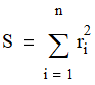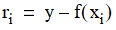# NMath User's Guide

Chapter 32. Nonlinear Least Squares (.NET, C#, CSharp, VB, Visual Basic, F#)

NMath provides classes for solving nonlinear least squares problems.

Solving a nonlinear least squares problem means finding the best approximation to vector y with the model function that has nonlinear dependence on variables x, by minimizing the sum, S, of the squared residuals:whereUnlike the linear least squares problem, non-linear least squares does not have a closed form solution, and is therefore solved by iterative refinement.

NMath provides nonlinear least squares classes for:

solving nonlinear least squares problems, with or without linear boundary constraints, using the Trust-Region or Levenberg-Marquardt methods

curve fitting, by finding a minimum in the curve parameter space in the sum of the squared residuals with respect to a set of data points

surface fitting, by finding a minimum in the surface parameter space in the sum of the squared residuals with respect to a set of data points

This chapter describes how to use the nonlinear least squares classes.

Top

Top